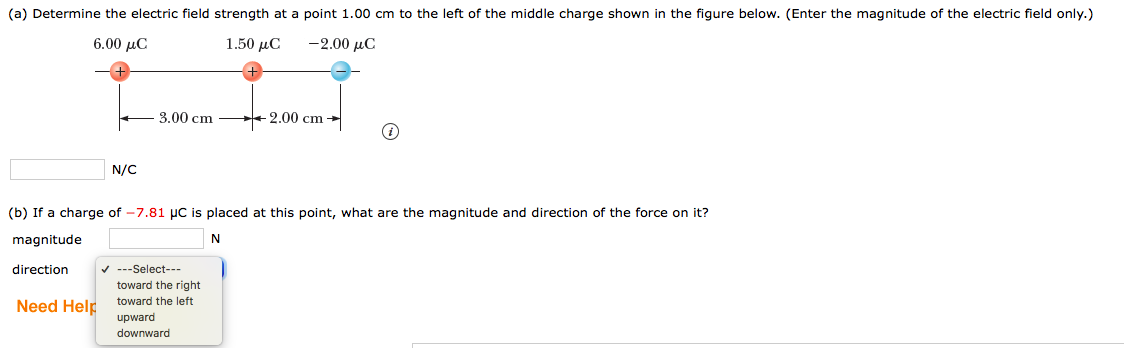# (a Determine the electric field strength at a point 1.00 cm to the left of the middle Charge shin the gure below.Enter the magnitude of the e ric eldon .6.00 μC1.50 μC-2.00 μC-3.00 cm2.00 cmN/C(b) If a charge of-7.81 μc is placed at this point, what are the magnitude and direction of the force on it?magnitude8V-Select--toward the rightNeed Hel toward the leftdownward

Question
4255 views

A. Determine the electric field strength at a point 1.00 cm to the left of the middle charge shown in the figure below. (Enter the magnitude of the electric field only.)

B.If a charge of −7.81 µC is placed at this point, what are the magnitude and direction of the force on it?help_outlineImage Transcriptionclose(a Determine the electric field strength at a point 1.00 cm to the left of the middle Charge sh in the gure below. Enter the magnitude of the e ric eldon . 6.00 μC 1.50 μC -2.00 μC -3.00 cm 2.00 cm N/C (b) If a charge of-7.81 μc is placed at this point, what are the magnitude and direction of the force on it? magnitude 8 V-Select-- toward the right Need Hel toward the left downward fullscreen
check_circle

Step 1

(a) Electric field due to charge q1 = 6.00μC is towards right. Electric field due to charge q2 = 1.50μC is towards left. Electric field due to charge q3 = -2.00μC is towards right.The net electric field at the point 1.00 cm to the left of middle charge is,

Step 2

(b) Force F on the charge q = -...

### Want to see the full answer?

See Solution

#### Want to see this answer and more?

Solutions are written by subject experts who are available 24/7. Questions are typically answered within 1 hour.*

See Solution
*Response times may vary by subject and question.
Tagged in

### Electric Charges and Fields